• 검색 결과가 없습니다.

# Overcoming Thermodynamic Limit of Subthreshold Swing in MOSFET:

N/A
N/A
Protected

Share "Overcoming Thermodynamic Limit of Subthreshold Swing in MOSFET: "

Copied!
131
0
0
더 보기 ( 페이지)

전체 글

50 Figure 4.4 (a) 3-dimensional view of EO resistance in an insulating pillar step structure, which is not necessarily located on the same material. 87 Figure A.1 (a) energy diagram of single level channel and (b) energy diagram of widened energy level channel due to coupling of contact.

Band theory

## Silicon

Material properties

### Carrier concentration

In the case of the n-type Si, carrier concentration in the thermal equilibrium is approximately given by. In the case of the p-type Si, carrier concentration in the thermal equilibrium is approximately given by.

## MOSFET characteristics

### Space charge on MOS

In the electric field expression (2.30), the first bracket describes the dependence on holes and ionized donors, and the second bracket describes the dependence on electrons and ionized acceptors.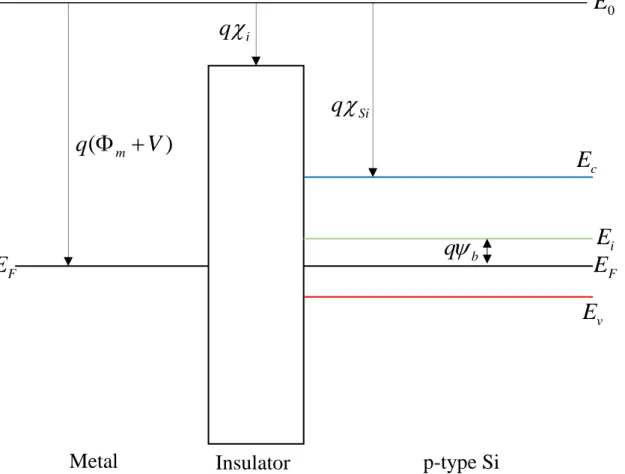Figure 2.3 Energy band diagram of MOS structure with p-type Si at equilibrium, flat band is induced with applied voltage equal to the difference between the work function of the metal and

### Diffusion current and the form of subthreshold swing

Where Vi C Qi d is the potential drop across the insulator and Ci id is the insulator capacitance. Another way is to achieve a negative insulator capacitance by using a ferroelectric material.

## Introduction

### Drain induced barrier lowering

The MOSFET structure is the heart of the logic circuits, and most electrical calculations are performed with MOSFET-based computer systems. This chapter describes the current challenges of nanometer-scale MOSFETs and proposes the solution for structural approach with a new structure called Edge-over MOSFET.

Subthreshold swing

## Suppressing subthreshold swing on transistor

### Suppressing subthreshold swing and drain induced barrier lowering in conventional

As shown in Figure 3.10, the calculated curve and the actual measurement curve (Figure 3 in ) are close and comparable . Moreover, the OFF state current of planar MOSFET is much larger than that of EO MOSFET, as shown in Figure 3.12(b).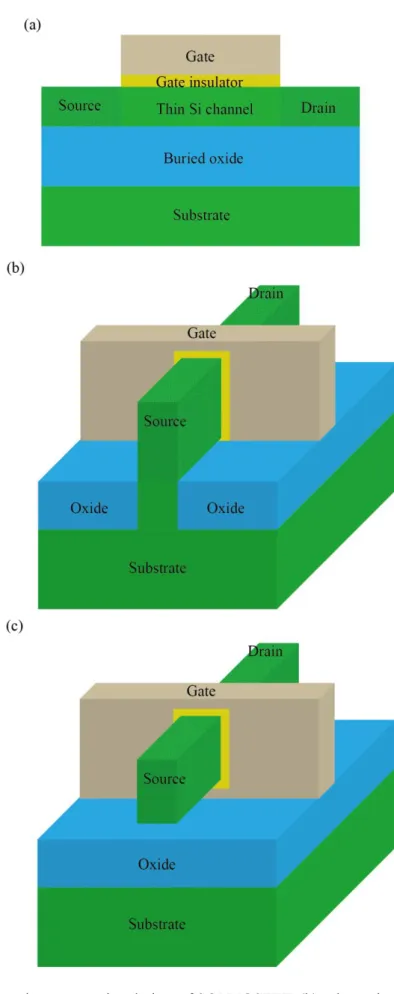Figure 3.2 (a) Schematic cross-sectional view of SOI MOSFET, (b) schematic view of FinFET, and (c) gate-all-around MOSFET

Suppressing subthreshold swing on various structural approaches

## Edge-over MOSFET for achieving thermodynamic limit of subthreshold swing

• Method and procedure
• Electrical characteristics
• Energy band and carrier concentration
• Conclusion

A schematic of the TCAD modeling process in the case of an EO MOSFET is shown in Figure 3.8. In TCAD modeling, the size of the transistor channel in the lateral dimension is 9.5 nm, as shown in Figure 3.9(a), at room temperature for the EO MOSFET and planar MOSFET. Vds, source-drain current vs. drain voltage) of the EO MOSFET with a pillar height of 36 nm and the planar MOSFET for the gate drive voltages (Vg −Vt) are shown in Fig. 3.12(a).

As the height of the insulation column is varied, the transfer characteristics of the EO MOSFET change at a drain bias of 0.5 V, as shown in Figure 3.13. Considering the material properties, the operational characteristics of EO MOSFET can be influenced by the grain boundaries of the poly-Si. The OFF-state energy band profiles along the transistor channel of the EO MOSFET and the planar MOSFET are shown in Figure 3.17(a) for NMOSFET at a drain bias of 0.5 V and in Figure 3.17(b) for PMOSFET at a drain bias of - 0.5 V .

The channel energy band diagram of EO MOSFET and planar MOSFET in the case of (a) NMOSFET and (b) PMOSFET .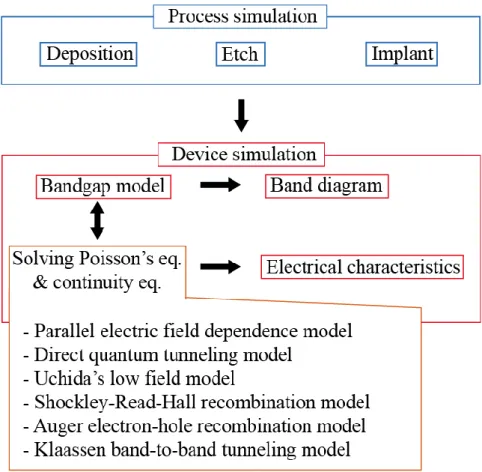Figure 3.8 TCAD modeling process of the EO MOSFET, device simulation is performed on the structural results of the process simulation

## Introduction

### Ternary logic circuit

In this chapter, the ternary converter composed of EO MOSFETs and two resistors on an integrated circuit is described with the operational characteristics obtained from TCAD mixed mode. In addition, the lowest complexity for binary logic circuit is the ternary logic circuit case in the second point of view. In summary, both perspectives explained the reduction of complexity from binary logic circuit to ternary logic circuit.

Since the number of logic cells and the complexity of the interconnections are reduced with the ternary logic circuit, the energy consumption of the entire system is reduced [ 40 - 44 ]. In recent decades, great progress has been made in the research into ternary logic operation and the ternary logic system. In the MOSFET, the technique of band-to-band tunneling in the OFF state, which is undesirable in conventional CMOS technology, creates the ternary state in the CMOS inverter .

In the case of a ternary circuit that uses additional electronic components with a conventional transistor, the ternary CMOS requires additional dimensions compared to the binary CMOS, so the higher information density in the surface cannot be guaranteed.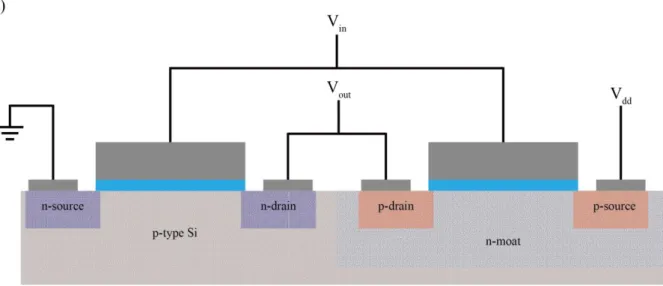Figure 4.1 (a) Schematic cross-sectional view of a conventional binary CMOS inverter with planar MOSFETs, and (b) Circuit diagram of relevant CMOS inverter, CMOS consists of NMOSFET and

## Edge-over Ternary Inverter

• TCAD mixed mode methodology for EO ternary inverter
• Edge-over Ternary Inverter
• Procedure of EO ternary inverter structure
• Electrical characteristics
• Conclusion

Likewise, EO resistance is increased with insulating pillar as shown in Figure 4.4.(a), hence the EO resistance is formed on the upper surface of insulating pillar, the lower part is formed on the shallow trench insulation (STI) surface and vertical part formed. is formed on the side wall of the insulating pillar. Sixth, patterned 5nm thick Poly-Si is deposited to define channel of MOSFETs and resistors (Figure 4.5.vi). Seventh, poly-Si for the EO resistor is implanted with phosphorus and boron to activate resistance (Figure 4.5.vii).

Finally, Cr and Ru gate electrodes are formed on the HfO2 layer for NMOSFET and PMOSFET respectively (Figure 4.5.ix). However, the 0.2 μm transistor width example that shows the best SNM for Vdd and 0 in Figure 4.8 is almost binary characteristics in Vin-Vout and there is no noise tolerance in the intermediate state. The variation of the region of the third state with Vdd in Figure 4.9 is explained by the region of operation of the transistors near the threshold.

However, the butterfly curve of the worst resistor mismatch combination has low SNM, especially the third state of the mismatch case 160kΩ-240kΩ and 240kΩ-160kΩ is unreliable due to mixing with the second state, as shown in Figure 4.10(b).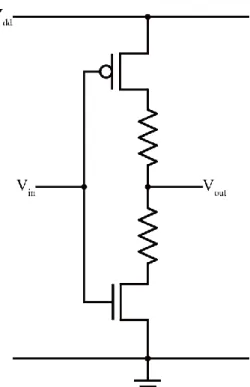Figure 4.2 Circuit diagram of ternary inverter consisted by two resistors, NMOSFET and PMOSFET

## Graphene

### Graphene Hamiltonian

A unique linear energy dispersion relation near the Dirac point yields a unique density of states proportional to energy. In 2004, the first experimental characterization of graphene was reported , and then a 3-dimensional Dirac semimetal was recently reported [52–55]. From the reciprocal vectors b1 and b2, it is possible to construct the first Brillouin zone of graphene in motion space, as shown in Figure 5.3.

Near the Dirac point D, which is the corners of the first Brillouin zone, Bloch Hamiltonian is approximated with relative vector to the Dirac point q k. Where, the Planck constant and vF is the fermi speed in graphene, which is extraordinarily high speed vF ~ / 300c (c is the speed of light). Graphene thus follows the linear energy distribution relation with fermi velocity and massless mechanics near the Dirac point.

In this regard, electrons near the Dirac point are called Dirac fermions and are described by the massless Dirac equation with the Pauli vector.

Graphene density of states near the Dirac point

## Thermionic emission at Graphene/Si interface

### Thermionic emission at Graphene/Si interface across the graphene layer

In the same approach as in (5.21), there are two different energy components, namely the vertical electron energy to the graphene layer Ex and the parallel electron energy along the graphene layer Ep . Unlike the linear energy dispersion along the graphene layer, the electron across the graphene layer in the perpendicular direction is treated with the conventional parabolic energy dispersion . In this way, the number of electrons across the graphene layer to Si is between the infinitesimal energy differences Ex ~ExdEx and E~EdE .

Schottky barrier height qb is determined by difference between graphene fermi level and electron affinity of Si, qb EF qSi, and energy difference between the Dirac point qgr and fermi level of graphene EF is ED EF  q gr. Where, EF,S is the fermi level of Si, which is related to voltage bias, when the graphene fermi level is reference point, EF,SEF V, and subscript  means the carriers are injected directly. However, since k TB ED is always true in n-graphene case, thermionic emission current always exists in n-type graphene case.

Similarly, the thermionic current density across the p-graphene layer with respect to Si is equal (in the case of Figure 5.8.ii and Figure 5.8.iii).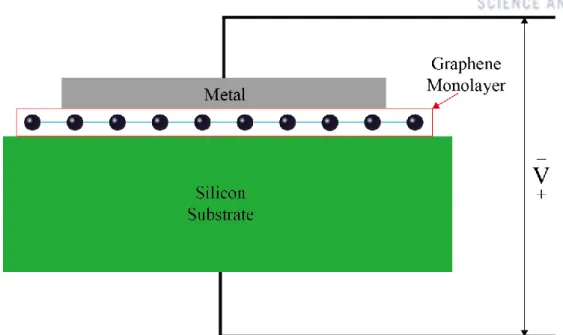Figure 5.6 Cross-sectional view of graphene/Si contact diode, electrons are injected into silicon across the graphene layer

### Thermionic emission at Graphene/Si interface without direct injection of carrier

It is evident that the thermionic current density of the p-graphene/Si interface has a term that depends linearly on the Schottky barrier height. In particular, the subthreshold oscillation of p-graphene/Si under the EDqb condition (Figure 5.8.iii) can break the thermodynamic limit, as a Dirac source of CNT FET . In Figure 5.10, the subthreshold oscillation diverges at EDqb, and the subthreshold oscillation is suppressed below the thermodynamic limit as ED qb approaches when ED qb.

Therefore, the conditions for ED and qb, which overcome the thermodynamic limit of subthreshold swing, always exist regardless of Schottky barrier height change on gate voltage  when the subthreshold current is dominated by thermionic emission. Meanwhile, the density of states for p-graphene decreases until the electron energy reaches the Dirac point, especially when the Schottky barrier height is located close to the Dirac point, thermionic emission is effectively suppressed due to the form of density of states and Fermi- Dirac statistics. Image power reduction to Schottky barrier height is included in depletion and inversion conditions, which describe the change of Schottky barrier height as maximum channel electric field near the metal/Si interface .

The simplified form of the Schottky barrier is modified by lowering the image power, as shown in Figure 5.12. Therefore, the tunnel current is underestimated by the simplified form of the Schottky barrier.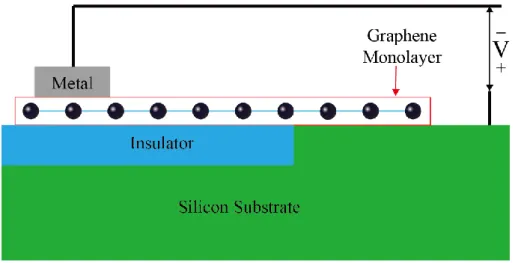Figure 5.9 Cross-sectional view of graphene/Si contact diode to prohibit the direct injection from graphene to Si

## Thermionic emission at 3 dimensional Dirac semimetal/Si interface without direct injection of

In this state, the subthreshold swing can break the thermodynamic limit with proper Schottky barrier change on gate voltage , as in the case of p-graphene/Si interface. Compared to graphene case, lowest subthreshold swing is given by theoretical, such as thermodynamic limit for conventional 3-dimensional bulk source with parabolic energy dispersion. Since the density of states of 3-dimensional Dirac semimetals increases as energy squared, the range of the difference between the fermi level and the Dirac point for the suppression of thermionic emission is actually wider than that of graphene.

The height of the Schottky barrier is adjusted by lowering the image force with a simplified setup as shown in Figure 5.12. Apparently, the thermionic emission current overrides the tunneling current under all conditions calculated with all gate biases. In the case of ED= 0.4 eV and ED= 0.6 eV, the smallest subthreshold fluctuations are estimated as 30 mV/dec and 41 mV/dec, especially 30 mV/dec is the lowest subthreshold slope as expected in the analytical approach.

Conclusion

## Non Equilibrium Green’s Function

### Non Equilibrium Green’s function (NEGF)

• Formalism of Non Equilibrium Green’s function (NEGF)
• Transmission and electric current with NEGF
• Electron-Phonon scattering formalism in NEGF

From (A.9), outflow of the channel is determined by self-energy  and wave function of the channel, as shown in (A.8), self-energy is described by coupling with energy and Hamiltonian of contact A (In theoretical physics, the energy change that occurs in the environment is called self-energy). Hamiltonian of the channel gives complex eigenenergy and eigenfunction due to self-energy of the channel. In the (A.28) with (A.29), imaginary part of self-energy describes the LDOS with Green's function, in this reason, called broadening function.

According to the self-consistent Born approximation, the self-scattering energy is described by the phonon propagator and the carrier correlation function as . The Fourier transformation of the self-scattering energy from the time interval t2−t1 into the energy E in the steady state gives Consequently, the intrinsic scattering energy of the inflow and outflow in the unbounded transverse direction is k (= .kt).

At the channel boundary (n=1 or N), since the self-energy gives the output waves at the boundaries as defined in the previous section (or the self-energy can be estimated by solving the surface Green's function .. of the source and the drain itself - consistently), the Bloch equation becomes .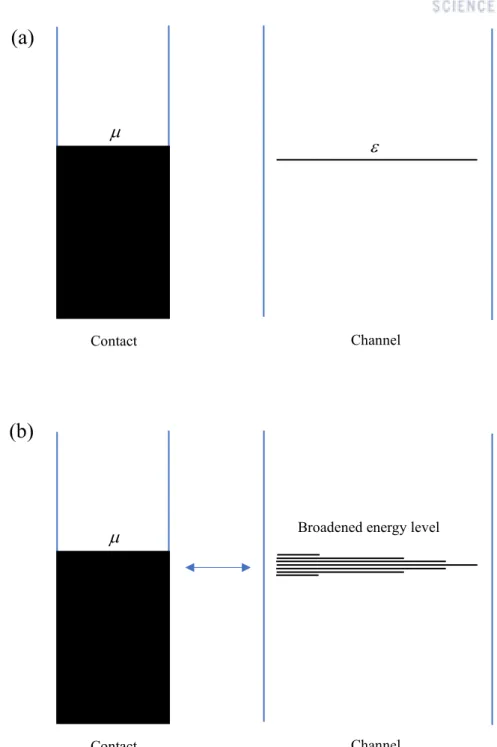Figure A.1 (a) energy diagram of single level channel and (b) energy diagram of broadened energy level channel due to coupling of contact

NEGF approach to 1 dimensional channel SOI MOSFET

• Self-consistent NEGF calculation with Poisson’s equation
• NEGF calculation for 1 dimensional channel MOSFET

수치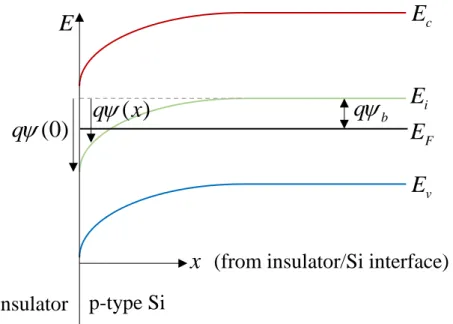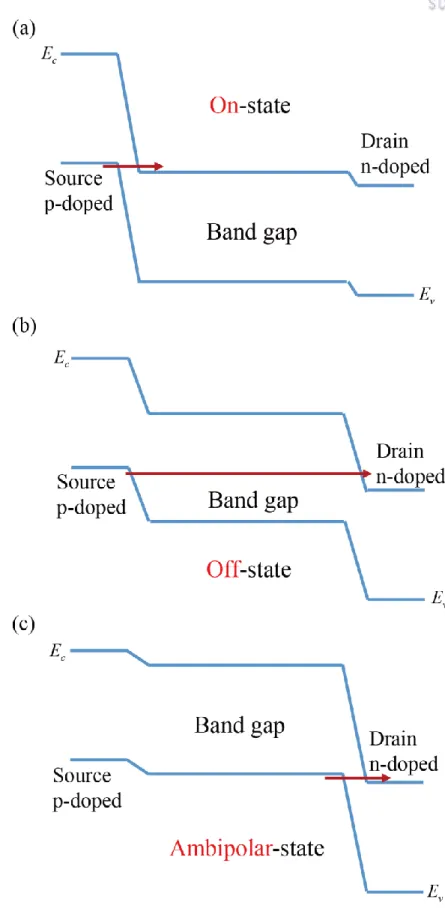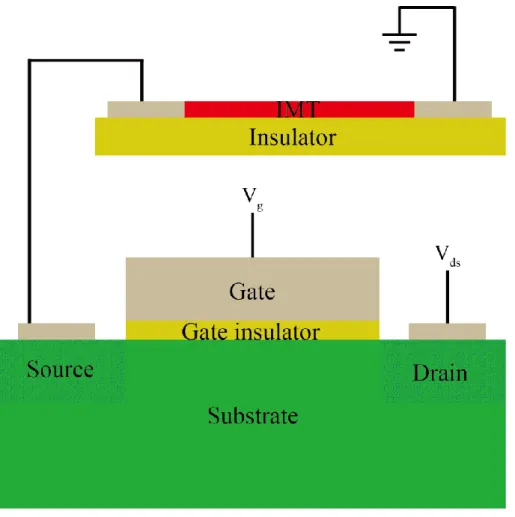+7

참조

관련 문서

The dome stretching tests and tension tests have been performed to obtain a forming limit curve(FLC) for the copper alloy which is used for manufacturing

Thermal limit, that is the rocked time which the motor can endure, is reversely propostional to the square of starting current.. Namely, when applying star-delta starting, the

 As a result, a residual drain current (which is called the subthreshold current) can flow between the source and drain.  The subthreshold current

Similar large quenching constants were also reported for the other quinolone antibiotics such as OFL and FLU.19 Usually large k q beyond the diffusion-controlled limit

• Adsorption isotherm: equilibrium data at a constant temperature - A limit on the extent to which a solute is adsorbed from a specific fluid.. mixture on a given adsorbent for

Although a microstrip slot antenna with a T-shaped feedline is capable of matching the input impedance for narrow as well as wide slot, it has the limit

Whereas intensive industrialization refers to an increase of manufacturing value added based on drivers that strengthen manufacturing industries (in terms of

The Eurostat online data code, which is given at the bottom of each column of data in the tables and as part of the source for figures, provides users with a quick and efficient

Such doubts about the global economy, the MOMR added, were “causing a great deal of uncertainty for the forecast for world oil demand, which has a downward risk, especially in

The Eurostat online data code, which is given at the bottom of each column of data in the tables and as part of the source for figures, provides users with a quick

언어 선택하기

웹사이트는 선택한 언어로 번역됩니다.

기타 언어